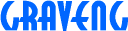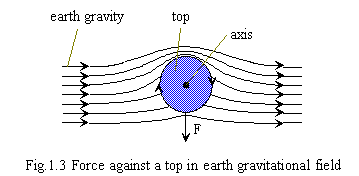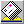1.6 Why a top does move as precession

A gravitation field is a field which can be superposed. For example, there are two planets 'A' and 'B', and have the same size and mass. There is zero gravity at point 'P' which is the intermediate point between planet 'A' and 'B'. This is the principle of superposition: gravity can be cancelled at the certain point between two planets.

A rotating gravitation field being synchronized with a spinning top surely exists. There are two gravitation fields around the top within the earth's gravitation field space: the earth's gravitation field and a rotating gravitation field. Given that it possible to superpose the gravitation field on another gravitation field, it should also be possible to superpose the rotating gravitation field on the earth's gravitation field.

You can imagine what would happen if some fields were superposed, recalling our consideration of 'Why a rotating ball curves'. The reason for a top's precession is similar to the curving of a rotating ball. A top maintains a stable rotation if its axis is parallel to the line of gravitation (the top's axis points to the core of the earth). For the same reason, a bullet maintains a straight line eventhough it's spinning.

However, there are different reactions occurred, in case of fluid like air flow,the force effected into the position where is amplified, but in case of gravitation is completely opposite. (Fig.1.3)It is well known that gravity can accelerate an object. An apple that drops from a tree is pulled by gravity. Acceleration corresponds to direction of gravity. We know that a top turns on a pivot as per fig.1.1 A top will follow the direction of the superposed gravitation field. The confined gravitation field direction around top is changed, it's not from up to down. We know that a top can vary the principle that gravity should be from up to down, which therefore means it is capable of changing the direction of the earth's gravitation field.

Next, to let consider about how to drive forward straight using rotating gravitation of like a spinning top in out of other gravitation field such as earth. Let's start by using hydraulic fluid as an example. Can propulsion be caused by fluid hydrodynamics. It would be similar to a rotating ball being curved by the surrounding differential pressure around a ball. Is there any method propelling straight using fluid hydrodynamics? You can't use propel. Propel is to compress air which means an application of reaction, this is not lift performance.

• Hint 1 ; Wing of airplane
• Hint 2 ; Boomerang
• Hint 3 : Frisbee
Do you know this? [The answer]

Updated 10/Oct/1996redsky@graveng.jp